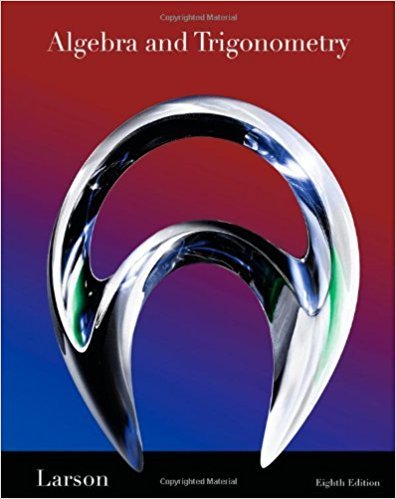×
×

# Solutions for Chapter 10.2: Operations with Matrices## Full solutions for Algebra and Trigonometry | 8th Edition

ISBN: 9781439048474Solutions for Chapter 10.2: Operations with Matrices

Solutions for Chapter 10.2
4 5 0 272 Reviews
13
4
##### ISBN: 9781439048474

Since 94 problems in chapter 10.2: Operations with Matrices have been answered, more than 49319 students have viewed full step-by-step solutions from this chapter. Chapter 10.2: Operations with Matrices includes 94 full step-by-step solutions. This expansive textbook survival guide covers the following chapters and their solutions. This textbook survival guide was created for the textbook: Algebra and Trigonometry, edition: 8. Algebra and Trigonometry was written by and is associated to the ISBN: 9781439048474.

Key Math Terms and definitions covered in this textbook
• Back substitution.

Upper triangular systems are solved in reverse order Xn to Xl.

• Big formula for n by n determinants.

Det(A) is a sum of n! terms. For each term: Multiply one entry from each row and column of A: rows in order 1, ... , nand column order given by a permutation P. Each of the n! P 's has a + or - sign.

• Cross product u xv in R3:

Vector perpendicular to u and v, length Ilullllvlll sin el = area of parallelogram, u x v = "determinant" of [i j k; UI U2 U3; VI V2 V3].

• Cyclic shift

S. Permutation with S21 = 1, S32 = 1, ... , finally SIn = 1. Its eigenvalues are the nth roots e2lrik/n of 1; eigenvectors are columns of the Fourier matrix F.

• Determinant IAI = det(A).

Defined by det I = 1, sign reversal for row exchange, and linearity in each row. Then IAI = 0 when A is singular. Also IABI = IAIIBI and

• Diagonalizable matrix A.

Must have n independent eigenvectors (in the columns of S; automatic with n different eigenvalues). Then S-I AS = A = eigenvalue matrix.

• Dimension of vector space

dim(V) = number of vectors in any basis for V.

• Free columns of A.

Columns without pivots; these are combinations of earlier columns.

• Full column rank r = n.

Independent columns, N(A) = {O}, no free variables.

• Hilbert matrix hilb(n).

Entries HU = 1/(i + j -1) = Jd X i- 1 xj-1dx. Positive definite but extremely small Amin and large condition number: H is ill-conditioned.

• Iterative method.

A sequence of steps intended to approach the desired solution.

• Nullspace matrix N.

The columns of N are the n - r special solutions to As = O.

• Nullspace N (A)

= All solutions to Ax = O. Dimension n - r = (# columns) - rank.

• Orthogonal subspaces.

Every v in V is orthogonal to every w in W.

• Projection matrix P onto subspace S.

Projection p = P b is the closest point to b in S, error e = b - Pb is perpendicularto S. p 2 = P = pT, eigenvalues are 1 or 0, eigenvectors are in S or S...L. If columns of A = basis for S then P = A (AT A) -1 AT.

• Rayleigh quotient q (x) = X T Ax I x T x for symmetric A: Amin < q (x) < Amax.

Those extremes are reached at the eigenvectors x for Amin(A) and Amax(A).

• Similar matrices A and B.

Every B = M-I AM has the same eigenvalues as A.

• Special solutions to As = O.

One free variable is Si = 1, other free variables = o.

• Spectral Theorem A = QAQT.

Real symmetric A has real A'S and orthonormal q's.

• Standard basis for Rn.

Columns of n by n identity matrix (written i ,j ,k in R3).

×